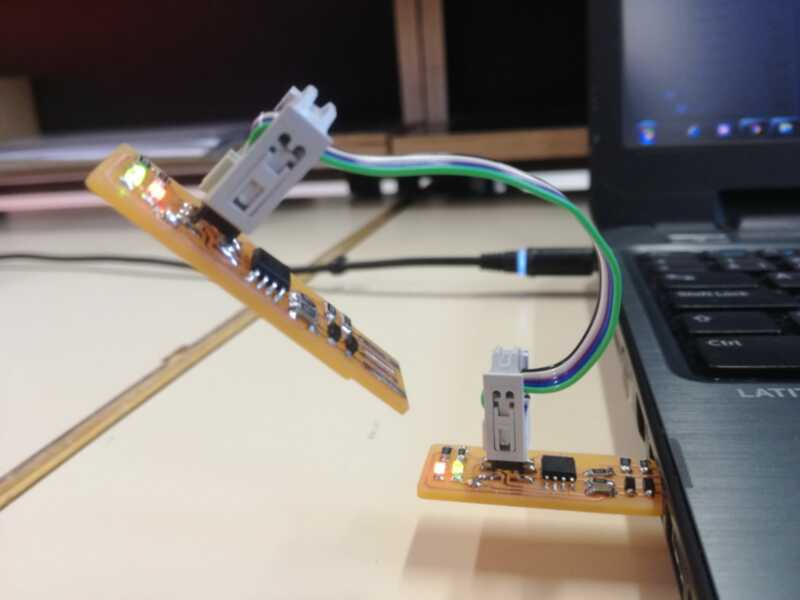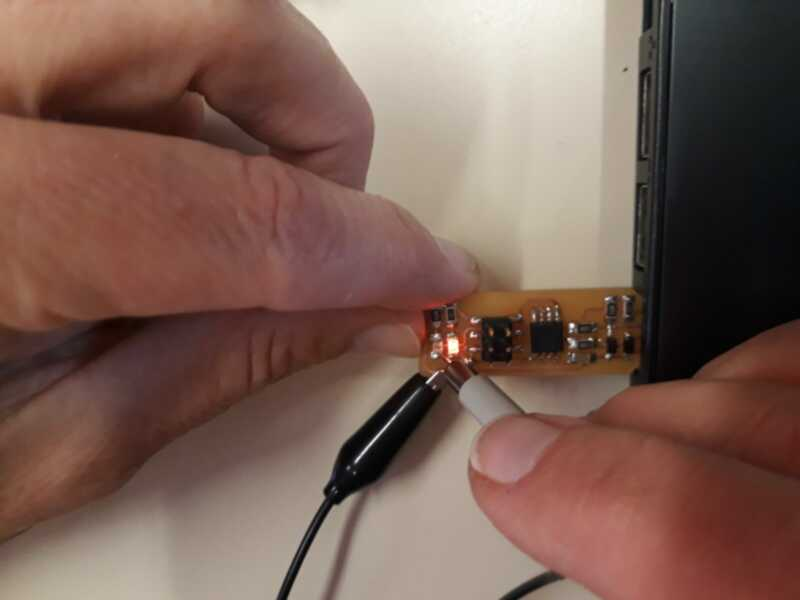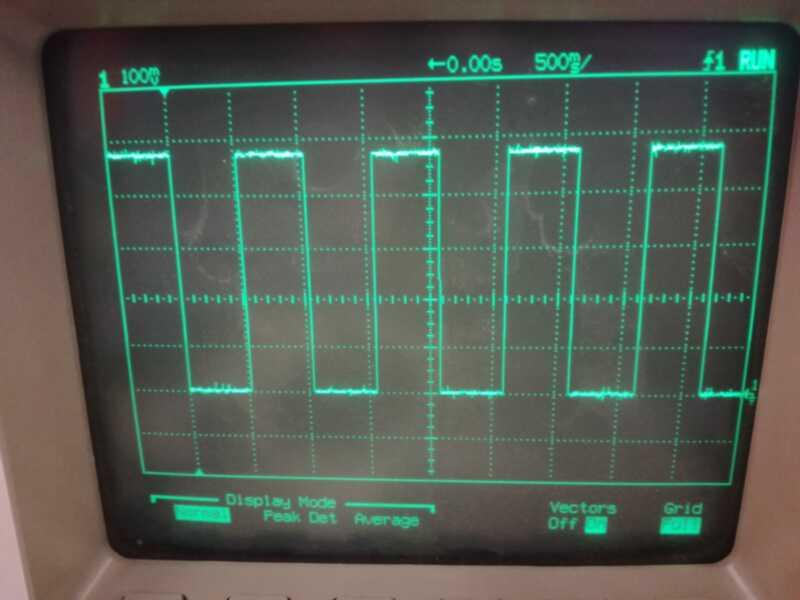# Electronic Design¶

## Group assignment:¶

Use the test equipment in your lab to observe the operation of a microcontroller circuit board

## Result¶

The minimum test equipment for a electronics lab consists generally in :

• regulated power supply

• low frequency generator

• oscilloscope

• logical analyzer

• multimeter

Resources available @FablabULB:

• HP 54602B oscilloscope, with HP 10071A 10:1 probes (attenuation ratio of 10:1: this means that the signal displayed on the screen is reduced by a factor 10)

• HAMEG Digital multimeter HM8011-3

As the lab is not completely equipped yet, we decided to go for simple measurements:

• Continuity check: with simple multimeter, we can check if there are shorts and no-contact on the nets (unpowered system).

• Diode check: with a simple multimeter, we can check if the diodes (Leds, Zener, signal, etc..) are polarized correctly (unpowered system)

• Voltage measurements: DC voltages at given points are easy to measure with a simple multimeter, AC voltages and time-varying signals are best measured with a oscilloscope…(powered system)

• Current measurements: more difficult to measure, as the loop where it flows needs to be opened....

We measure the signal on VCC pin: (either on the multimeter or the oscilloscope)

We measure the signal on the blinking led :programming measuring

To see the variations, we best use the oscilloscope:We recognize the 1sec period (scale is 500msec/square), and the attenuation factor of 10 (scale is 100mV/square)